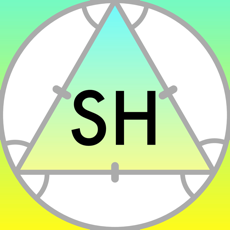School HelperDeveloped by a high school student going through geometry class, School Helper is a useful tool that can save you time and improve your grade in geometry class. School Helper is a combination of math tools that allow you to complete your work quickly and accturately.

Features

Trigonometry Solver

The most simple and quick trigonometry solver, capable of solving:

• Side-Side-Side (SSS) triangles
• Size-Angle-Size (SAS) triangles
• Angle-Size-Angle (ASA) triangles
• Angle-Angle-Size (AAS) triangles
• Size-Side-Angle (SSA) triangles

Volume and Surface Area Solver

Solve the volume and surface area for different 3D shapes including:

• Sphere
• Cylinder
• Cone
• Rectangular prism
• Triangular prism
• Pyramid

Circles and Arcs

Calculate arc length and sector area of a circle given angle and radius. Choose between degrees and radians for your calculation.

Polygon Perimeter and Area (Chrome only)

Enter the radius and number of sides to calculate the perimeter and area.

Availability

School Helper is a useful tool. Many students use a Chromebook today so that prompted us to release school helper for Chrome extension. School Helper is available in an iOS app and a Chrome extension.

Contact

Reach out to the creator: Nick.